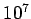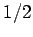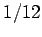Next: C++ extensions to C Up: Scientific programming in C Previous: Timing

## Random numbers

A large class of scientific calculations (e.g., so-called Monte Carlo calculations) require the use of random variables. A call to the rand() function (header file stdlib.h), with no arguments, returns a fairly good approximation to a random integer in the range 0 to `RAND_MAX` (defined in stdlib.h). The srand() function sets its argument, which is of type int, as the seed for a new sequence of numbers to be returned by rand(). These sequences are repeatable by calling srand() with the same seed value. If no seed value is provided, the rand() function is automatically seeded with the value 1. It is common practice in C programming to seed the random number generator with the number of seconds elapsed since 00:00:00 UTC, January 1st, 1970. This number is returned, as an integer, via a call to the time (NULL) function (header file: <time.h>). Seeding the generator in this manner ensures that a different set of random numbers is generated automatically each time the program is run.

The program listed below illustrates the use of the rand() function to construct a pseudo-random variable, x, which is uniformly distributed in the range 0 to 1. The program calculatesvalues of x, and then evaluates the mean and variance of these values.

```
/* random.c */
/*
Program to test operation of rand() function
*/

#include <stdlib.h>
#include <stdio.h>
#include <time.h>
#define N_MAX 10000000

int main()
{
int i, seed;
double sum_0, sum_1, mean, var, x;

/* Seed random number generator */
seed = time(NULL);
srand(seed);

/* Calculate mean and variance of x: random number uniformly
distributed in range 0 to 1 */
for (i = 1, sum_0 = 0., sum_1 = 0.; i <= N_MAX; i++)
{
x = (double) rand() / (double) RAND_MAX;

sum_0 += x;
sum_1 += (x - 0.5) * (x - 0.5);
}
mean = sum_0 / (double) N_MAX;
var = sum_1 / (double) N_MAX;

printf("mean(x) = %12.10f  var(x) = %12.10f\n", mean, var);

return 0;
}
```
The typical output from this program is as follows:
```
mean(x) = 0.5000335261  var(x) = 0.0833193874
%
```
As is easily demonstrated, the theoretical mean and variance of x areand, respectively. It can be seen that the values returned by the program agree with these theoretical values to five decimal places, which is all that can be expected with onlycalls.Next: C++ extensions to C Up: Scientific programming in C Previous: Timing
Richard Fitzpatrick 2006-03-29